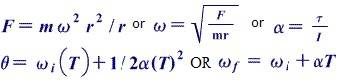# Rotational Dynamics

## Homework Statement

A 10kg objects rotates on a point on a wire 3 meters long at the rate of 120 rad/sec without breaking the wire.

1) What is the numeric value (and units) of the velocity vector and 2)what angle does it make with the tangent at any point? and 3) What's the minimum tensile strength of wire asumming that is has a 50% safety factor? (Minimum tensile strength of the wire is 1.5 times the working tensile strength)

## Homework Equationsor V=ωr or θ=S/r

## The Attempt at a Solution

V=ωr
V=120(3)= 360 m/sec

α=v^2/r
α=129600/3
α= 43200

t= (Vf-Vi)/a
t=(360/43200)
t=.00833 seconds

theta=ω(t)
theta=120(.00833)= 1

Last edited:

Doc Al
Mentor

## The Attempt at a Solution

V=ωr
V=120(3)= 360 m/sec
That's the correct approach and answer for question 1.

α=v^2/r
α=129600/3
α= 43200
That's the centripetal acceleration. Now apply Newton's 2nd law to work out the tension.

t= (Vf-Vi)/a
t=(360/43200)
t=.00833 seconds

theta=ω(t)
theta=120(.00833)= 1
No clue what you are doing here.